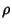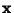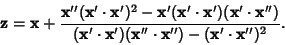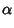## Curvature Center

The point on the Positive Ray of the Normal Vector at a distance, whereis the Radius of Curvature. It is given by(1)

whereis the Normal Vector andis the Tangent Vector. It can be written in terms ofexplicitly as(2)

For a Curve represented parametrically by,(3)(4)

References

Gray, A. Modern Differential Geometry of Curves and Surfaces. Boca Raton, FL: CRC Press, 1993.